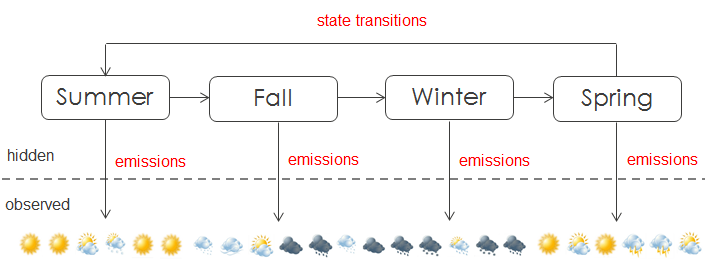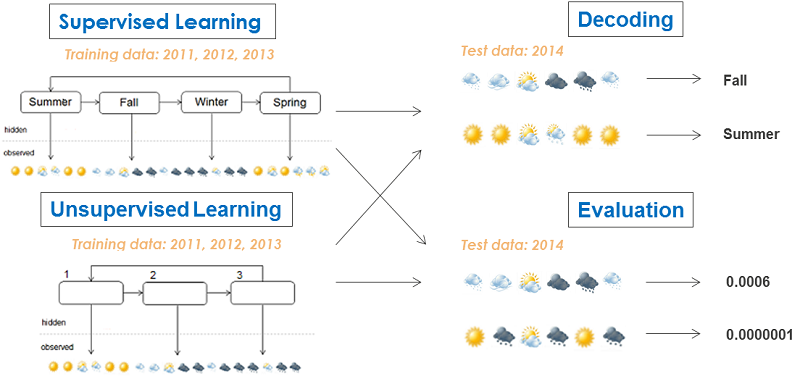# 1.1 - 8.10 - Introduction to Hidden Markov Models - Teradata Vantage

## Teradata Vantage™ - Machine Learning Engine Analytic Function Reference

Product
Release Number
1.1
8.10
Published
October 2019
Content Type
Programming Reference
Publication ID
B700-4003-079K
Language
English (United States)

The Hidden Markov model is a statistical model that describes the evolution of observable events that depend on internal factors that are not directly observable. The following figure shows the key elements of the model.

The figure has two parts, divided by a dashed line. Below the dashed line is the observed sequence; above the dashed line are the hidden states (so called because they are not directly observable). The hidden states have state transitions that introduce the state sequences.

In the following figure, the observed sequence is the weather, the hidden states are the seasons, and the state sequence is summer, fall, winter, spring. The states have outgoing edges to the observations, where the edge represents the emission. For example, summer emits good weather and winter emits bad weather.The HMM model addresses three problems: Learning, Decoding, and Evaluating. The following figure assumes that historical weather data from years 2011 to 2013 is available to train the model. If the hidden states are labeled in the training data, this type of training process is called supervised learning. Otherwise, it is called unsupervised learning. To use unsupervised learning, you must specify the number of hidden states. After the model is trained, you can make predictions. Given the observed sequence, inferring the internal state is called decoding. Given the sequence, measuring the probability of the sequence is called evaluation.## HMM Models, Descriptions, and ML Engine Functions

HMM Model Description ML Engine Function
Unsupervised Learning Given observation sequence and number of states, find model that maximizes probability of observed sequence. HMMUnsupervised (ML Engine)
Supervised Learning Given observation sequences and states, find model that maximizes probability of observed sequence. HMMSupervised (ML Engine)
Evaluation Given trained model and observation sequence, find probability of sequence. HMMEvaluator (ML Engine)
Decoding Given trained model and observation sequence, find optimal state sequence. HMMDecoder (ML Engine)